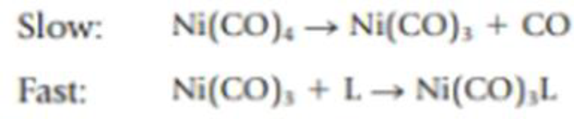Chapter 14, Problem 88IL

Chapter
Section
Textbook Problem

The substitution of CO in Ni(CO)4 by another molecule L [where L is an electron-pair donor such as P(CH3)3] was studied some years ago and led to an understanding of some of the general principles that govern the chemistry of compounds having metal-CO bonds. (See J. P. Day, F. Basolo, and R. G. Pearson: Journal of the American Chemical Society, Vol. 90. p. 6927, 1968.) A detailed study of the kinetics of the reaction led to the following mechanism:(a) What is the molecularity of each of the elementary reactions? (b) Doubling the concentration of Ni(CO)4 increased the reaction rate by a factor of 2. Doubling the concentration of L had no effect on the reaction rate. Based on this information, write the rate equation for the reaction. Does this agree with the mechanism described? (c) The experimental rate constant for the reaction, when L = P(C6H5) is 9.3 × 10−3 s−1 at 20 °C. If the initial concentration of Ni(CO)4 is 0.025 M, what is the concentration of the product after 5.0 minutes?

(a)

Interpretation Introduction

Interpretation: The molarity of each of the elementary reactions should be determined.

Concept introduction:

Molecularity: It is defined as the number of reacting species involved in a chemical reaction.

Explanation

Molecularity of a chemical reaction is obtained by considering the number of reacting species involved in it.

The reaction is give below:

Slow: Ni(CO)4Ni(CO)3+CO

(b)

Interpretation Introduction

Interpretation: Rate equation of the reaction should be determined and decided whether it agrees with the given mechanism.

Concept introduction:

Rate law or rate equation: Rate law:

It is generally the rate equation that consists of the reaction rate with the concentration or the pressures of the reactants and constant parameters.

aA + bBxXRate of reaction = k [A]m[B]n

Rate constant, k: The rate constant for a chemical reaction is the proportionality term in the chemical reaction rate law which gives the relationship between the rate and the concentration of the reactant present in the chemical reaction.

(c)

Interpretation Introduction

Interpretation: The concentration of the product should be determined after 5.0minutes.

Concept introduction:

Rate law or rate equation: Rate law:

It is generally the rate equation that consists of the reaction rate with the concentration or the pressures of the reactants and constant parameters.

aA + bBxXRate of reaction = k [A]m[B]n

The integrated rate law for the first order is as follows:

k=1tln[A0][A]Where,[A0]= initialconcentrationt=time[A]=concentrationaftertimet

Still sussing out bartleby?

Check out a sample textbook solution.

See a sample solution

The Solution to Your Study Problems

Bartleby provides explanations to thousands of textbook problems written by our experts, many with advanced degrees!

Get Started

Find more solutions based on key concepts